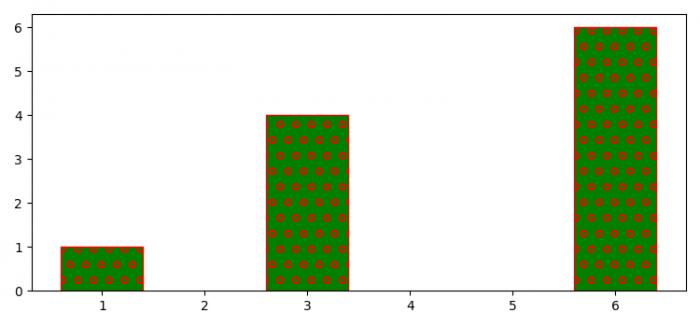# How to decrease the hatch density in Matplotlib?

To decrease the hatch density in Matplotlib, we can take the following steps −

• Set the figure size and adjust the padding between and around the subplots.

• Make a customized horizontal hatch class to override the density.

• Append the horizontal hatch class.

• Create a new figure or activate an existing figure.

• Add an 'ax1' to the figure as part of a subplot arrangement.

• Make lists of data points.

• Make a bar plot with x and ydata points, with hatch='o', color='green' and edgecolor='red'.

• To display the figure, use show() method.

## Example

from matplotlib import pyplot as plt, hatch

plt.rcParams["figure.figsize"] = [7.50, 3.50]
plt.rcParams["figure.autolayout"] = True

class MyHorizontalHatch(hatch.HorizontalHatch):
def __init__(self, hatch, density):
char_count = hatch.count('o')
if char_count > 0:
self.num_lines = int((1.0 / char_count) * density)
else:
self.num_lines = 0
self.num_vertices = self.num_lines * 2
super().__init__(hatch, density)

hatch._hatch_types.append(MyHorizontalHatch)

fig = plt.figure()

x = [3, 6, 1]
y = [4, 6, 1]

ax1.bar(x, y, color='green', edgecolor='red', hatch="o", lw=1., zorder=0)

plt.show()

## OutputUpdated on: 23-Sep-2021

342 Views